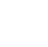Back to Chapter
Getting StartedBasic Syntax & DeclarationControl StatementsNo items found.
Function & EventsNo items found.
Array and its TypesNo items found.
OOP : Object Oriented ProgrammingNo items found.
Javascript StandardsNo items found.
HTML DOMNo items found.No items found.No items found.No items found.

# Introduction

Numbers in Javascript can be of 2 Types i.e numbers with decimals(Floating Pointers) and without decimals

Example : 2,3,4,5,6,... etc are numbers without decimals.

2.3, 4.5, 5.8,... etc are numbers with decimals.

``` var x = 2; var y = 4.5; ```

Syntax for Properties

``` Number.propertyName ```

Properties

Example

``` console.log(Number.MAX_VALUE); // Output // 1.7976931348623157e+308 ```

Syntax for Methods

``` Number.methodName(number) ```

Methods

Example

``` console.log(Number.isInteger(500)); console.log(Number.isInteger(2.5)); // Output // True // False ```